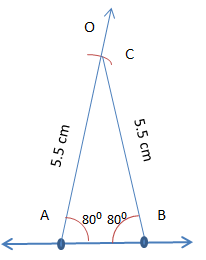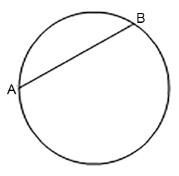Courses

# Points to Remember - Practical Geometry Class 8 Notes | EduRev

## Class 8 : Points to Remember - Practical Geometry Class 8 Notes | EduRev

The document Points to Remember - Practical Geometry Class 8 Notes | EduRev is a part of the Class 8 Course Class 8 Mathematics by VP Classes.
All you need of Class 8 at this link: Class 8

WE KNOW THAT
A triangle has six elements—3 sides and 3 angles. To construct a unique triangle, 3 elements out of six elements are required under a certain combination. A quadrilateral has 8 elements—4 sides and 4 angles. In addition to these elements a quadrilateral has 2 diagonals which play an important role in determining the size and shape of a quadrilateral. Thus a quadrilateral has 10 elements (4 sides, 4 angles and 2 diagonals) or measurements.

To construct a unique quadrilateral we need to know 5 measurements (elements).
Note: To construct a unique quadrilateral simply the knowledge of any five elements is not sufficient.
We will need to know a combination of specific 5 elements.

VARIOUS COMBINATIONS OF ELEMENTS FOR CONSTRUCTING A UNIQUE QUADRILATERAL
With the help of the following measurements we can construct quadrilaterals.
(i) Four sides and a diagonal.
(ii) Three sides and two diagonals.
(iii) Four sides and an angle.
(iv) Three sides and two included angles.
(v) Two adjacent sides and three angles.
(vi) Using special properties of a square or a rhombus, etc.

Solved Example:
Question: Suppose the two sides of the triangle are of the same length of 5.5 cm. One of the angles made by this side of the triangle is 80°. Find the other two angles. Construct the triangle.
Solution:
Since the two sides of the triangle are of the same length, the angles made by them with the base will always be the same. The angles made by them are of 80° each. By the property of the sum of the interior angles of a triangle, we have
x + 80° + 80° = 180° (let the third angle is of x degrees).
or, x = 180° – 160° = 20°.
The required triangleQuestion: What is the maximum number of regions into which a chord will divide a circle?
a. 1
b. 2
c. 3
d. 4
Solution:
2. The maximum number of regions into which a chord will divide a circle is 2, as AB is the chord which divides the circle into two regions.Question: Which of the following angle is possible to construct using a compass?
a. 60 °
b. 32 °
c. 51.25 °
d. 40 °
Solution:
A. 60 ° angle is possible to construct using a compass.

Question: To construct a line segment of a given length, which of the following pairs of instruments are needed?
a. Ruler and Protractor
b. Ruler and Compass
c. Compass and Divider
d. Protractor and Divider
Solution:
B. Ruler and Compass are used to construct a line segment.

Question: If PQ is the perpendicular bisector of AB then PQ divides AB in the ratio:
a. 1: 2
b. 1: 3
c. 2: 3
d. 1: 1
Solution:
D. The perpendicular bisector always divides the segment into 2 equal parts.

∴ PQ divides AB in the ratio 1: 1.

Offer running on EduRev: Apply code STAYHOME200 to get INR 200 off on our premium plan EduRev Infinity!

89 docs|16 tests

,

,

,

,

,

,

,

,

,

,

,

,

,

,

,

,

,

,

,

,

,

;